Math Concepts

# How can you discover decimals with cuemath?

316 views

 1 Introduction 2 So why do we use decimals? 3 The Cuemath Way of Solving decimals 4 Everyday uses of Decimals 5 Conclusion 6 About Cuemath 7 Frequently Asked Questions (FAQs) 8 External references

13 November  2020

## Introduction

In algebra, a decimal number can be defined as a number whose whole number part and the fractional part is separated by a decimal point. Our education system tells children how to manipulate and calculate decimals but they are never taught what decimals really are.

One day, we asked a group of grade 5 students whether 0.19 is greater than 0.2 or not. To our surprise, most of them said 0.19 is greater and the reason they gave was that 0.19 has 19 while 0.2 has 2.

## So why do we use decimals?

Decimals are used in situations which require more precision than whole numbers can provide. A good example of this is money: Three and one-fourth rupees is an amount between 3 rupees and 4 rupees. We use decimals to write this amount as Rs.3.25

A decimal may have both a whole-number part and a fractional part. The whole-number part of a decimal are those digits to the left of the decimal point. The fractional part of a decimal is represented by the digits to the right of the decimal point. The decimal point is used to separate these parts.

## The Cuemath Way of Solving decimals

When children learn decimals with Cuemath, they learn with the help of learning aids, children build a conceptual understanding to answer questions like these correctly.

Let’s take a 10*10 grid and think of it as 1 unit. Let’s call it 1 flat. Then let’s take a 10*1 column of this grid and call it 1 rod. Note that 1 rod has a value of 1/10 which we can write as 0.1.  Now, take a 1*1 grid such that 1 block equals 1/100. We can write it as 0.01.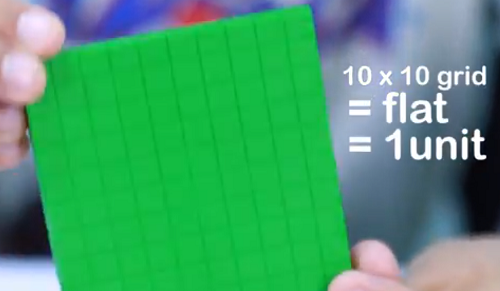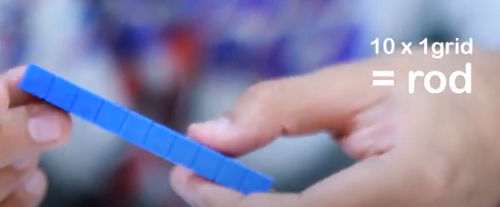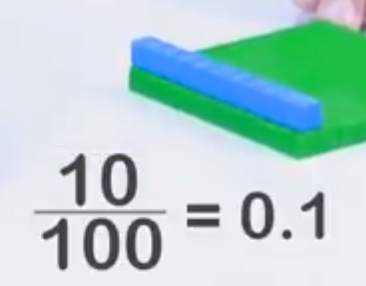With these modelling tools, it becomes easier for children to visualise decimals. They can represent a collection of flats, rods and blocks using decimal numbers. For example, one flat plus two rods is equal to 1.2. Similarly, they can represent 0.43 as the sum of 4 rods and 3 blocks.

Now let’s get back to our question of finding whether 0.19 is greater than 0.2 or not with the help these Base Ten Blocks. Now it is crystal clear that 0.2 is great than 0.19. Way simple than mugging formulae. Isn’t it?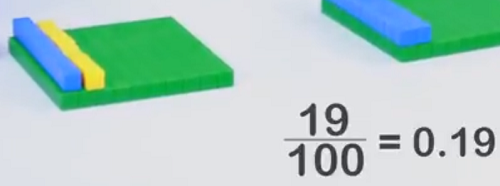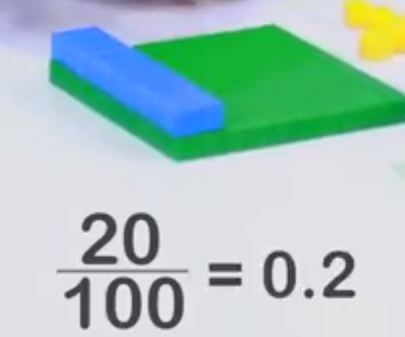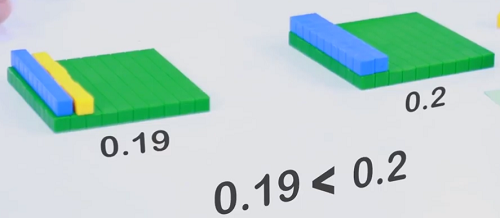Isn’t this a great way to learn decimals with Cuemath?

Your child will never forget a beautiful piece of mathematical reasoning like this. This academic session, let your child discover the new way of learning with Cuemath!

## Everyday uses of Decimals

It is inevitable dealing with decimal numbers when dealing with money. In many situations, such as when you have to convert paisa into rupee. Suppose you go to a local shop to buy 500 gm of tomato, where one kg of tomato costs Rs. 51. So, how much money should we hand over to the shopkeeper? We divide Rs. 51 by 2 which is equal to 25.5. In order to hand over the exact amount, we must understand what 25.5 means in terms of rupees where

• Re 1 = 100 paisa
• Re 0.5 = 50 paisa
• Rs. 25.5 = Rs 25 and 50 paisa

One more example is when you calculate your weight on the weighing machine, we do not always find the weight equal to a whole number on the scale. To know our exact weight, we must understand what the decimal value on the scale means.

## Conclusion

You can conclude by saying that a decimal is a combination of the whole number and a fraction of a whole number. A fraction can also be called as a mixed number can be turned into a decimal and vice versa. We use decimals in daily life while dealing with money, weight, length, etc.

Why does Cuemath have a different approach of explaining decimals?

Cuemath tries to explain all the concepts to its students in a similar fashion. Firstly, it uses practical examples to which students can relate to. Secondly, it breaks a numerical into different steps that are easy to understand. Finally, it provides students with enough assignments and numerical, so that they can undergo the regular practice. Remember, learning never stops.

Cuemath, a student-friendly mathematics and coding platform, conducts regular Online Live Classes for academics and skill-development, and their Mental Math App, on both iOS and Android, is a one-stop solution for kids to develop multiple skills.Understand the Cuemath Fee structure and sign up for a free trial.

## Why do we use decimals?

Decimals are used in situations which require more precision than whole numbers can provide.

## How to divide decimals

To divide decimal numbers: Multiply the divisor by as many 10's as necessary until we get a whole number. Remember to multiply the dividend by the same number of 10's.

## How to multiply decimals

To multiply decimals, first multiply as if there is no decimal. Next, count the number of digits after the decimal in each factor. Finally, put the same number of digits behind the decimal in the product.

## How to convert fractions to decimals

So, to convert a fraction to a decimal, divide the numerator by the denominator. If required, you can use a calculator to do this. This will give us our answer as a decimal.